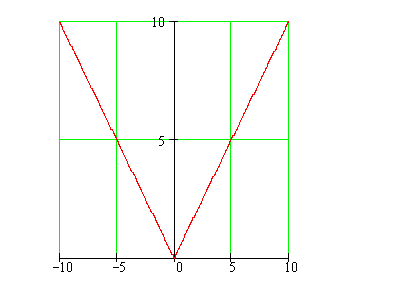Calculus on Demand at Dartmouth College Lecture 8 | Index | Lecture 10 Lecture 9Resources

Math 3 Course Syllabus
Practice Exams

Contents

In this lecture we continue the discussion of tangent lines, and define the derivative of a function at a point. (There should be drum rolls and much clapping in the background here. The definition of the derivative has been our goal from the first lecture.)

Quick Question

Does this function have a unique tangent line at x = 0?Outline

Outline for The Derivative

Textbook

Tangent Lines and their Slopes
The Derivative